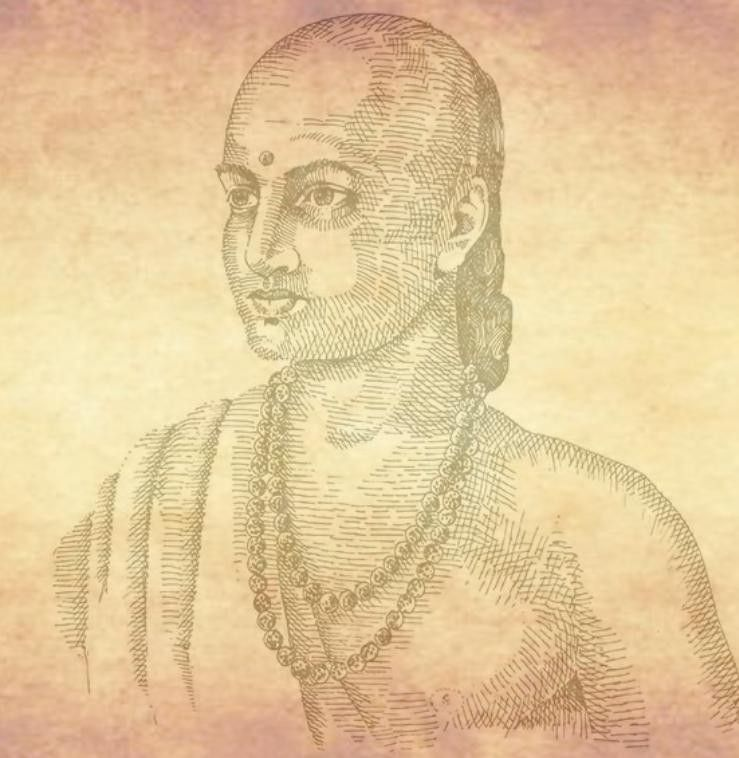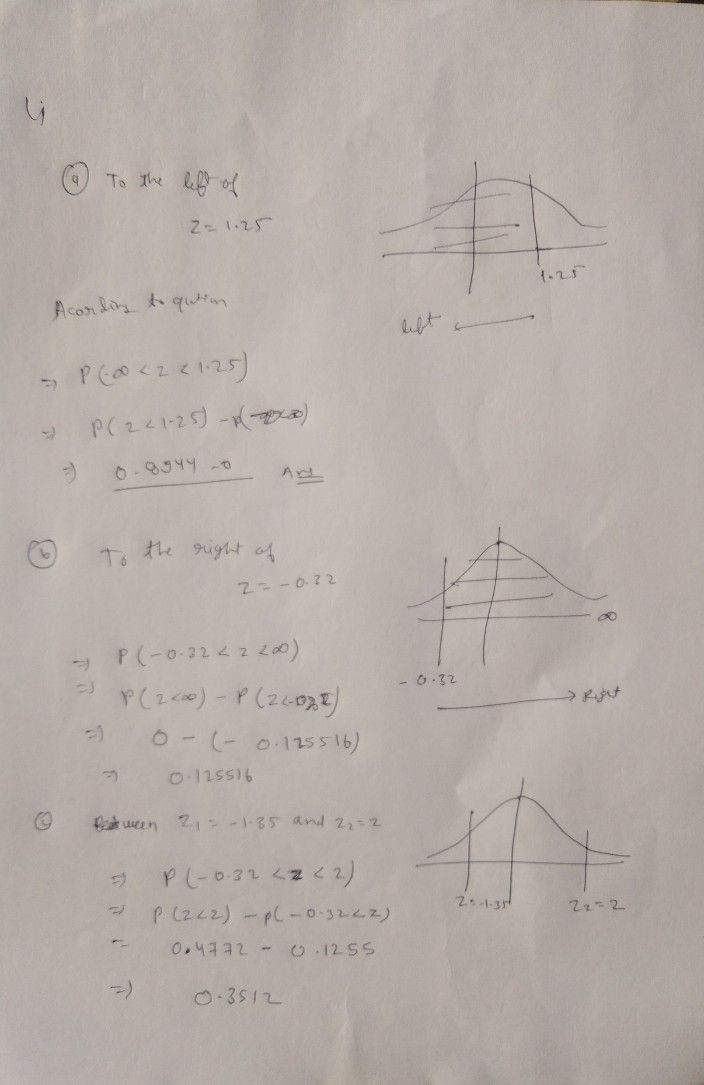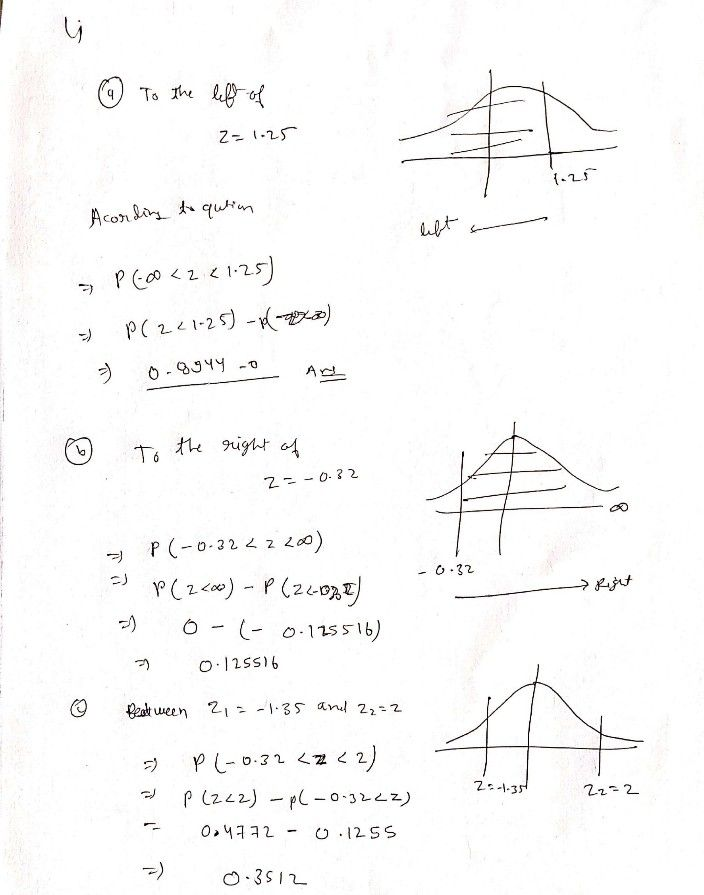Symbol
Problem$1.$ Find the area under the standard normal curve a) To the left of $z=1.25$ b) To the right of $z=-0.32$ c) Between $z_{1}=-1_{.35}$ and $z_{2}=2$
10th-13th grade
Probability and Statistics
SolutionQanda teacher - askBrij